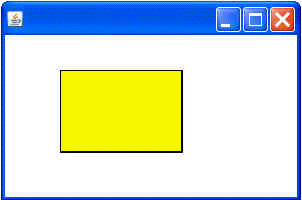Tutorials

# Rectangle Image in Java

In this section, you will learn how to create rectangle image. This program used the Graphics2D class which extends the Graphic class and control all geometry or coordinate transformation and color management, text layout.

In this section, you will learn how to create rectangle image. This program used the Graphics2D class which extends the Graphic class and control all geometry or coordinate transformation and color management, text layout.

# Rectangle Image in Java

Introduction

In this section, you will learn how to create rectangle image. This program used the Graphics2D class which extends the Graphic class and control  all geometry or coordinate transformation and color management, text layout. The Java programming in  java 2D API provides several classes. It defines that common  geometry object such as lining drawing , rectangle Drawing , curve drawing and Circle. All types of Geometry classes parts are  supported by java.awt.geom package.

Program Description:

In this program, you can also see how to used Rectangle2D for rectangle component. Define, first of all, class name for the rectangle component. The rectangle2D class describes a rectangle and is defined by the this location (x , y) coordinate and dimension (width , height).

Rectangle2D.Double(): This class is used to define a rectangle specified in double coordinate.

setPaint(): This component used for filling the color into the rectangle image.

Here is the code of this program:

 ``` import java.awt.*; import java.awt.event.*; import java.awt.geom.*; public class AWTRectangle extends Frame {   Stroke drawingStroke = new BasicStroke(3);   Rectangle2D rect = new Rectangle2D.Double(60, 70, 120, 80);      public void paint(Graphics g) {   Graphics2D g1 = (Graphics2D)g;   g1.setStroke(drawingStroke);   g1.draw(rect);   g1.setPaint(Color.yellow);   g1.fill(rect);   }   public static void main(String args[]) {   Frame frame = new AWTRectangle();   frame.addWindowListener(new WindowAdapter(){   public void windowClosing(WindowEvent we){   System.exit(0);   }   });   frame.setSize(300, 200);   frame.setVisible(true);   } }```

Output this program: